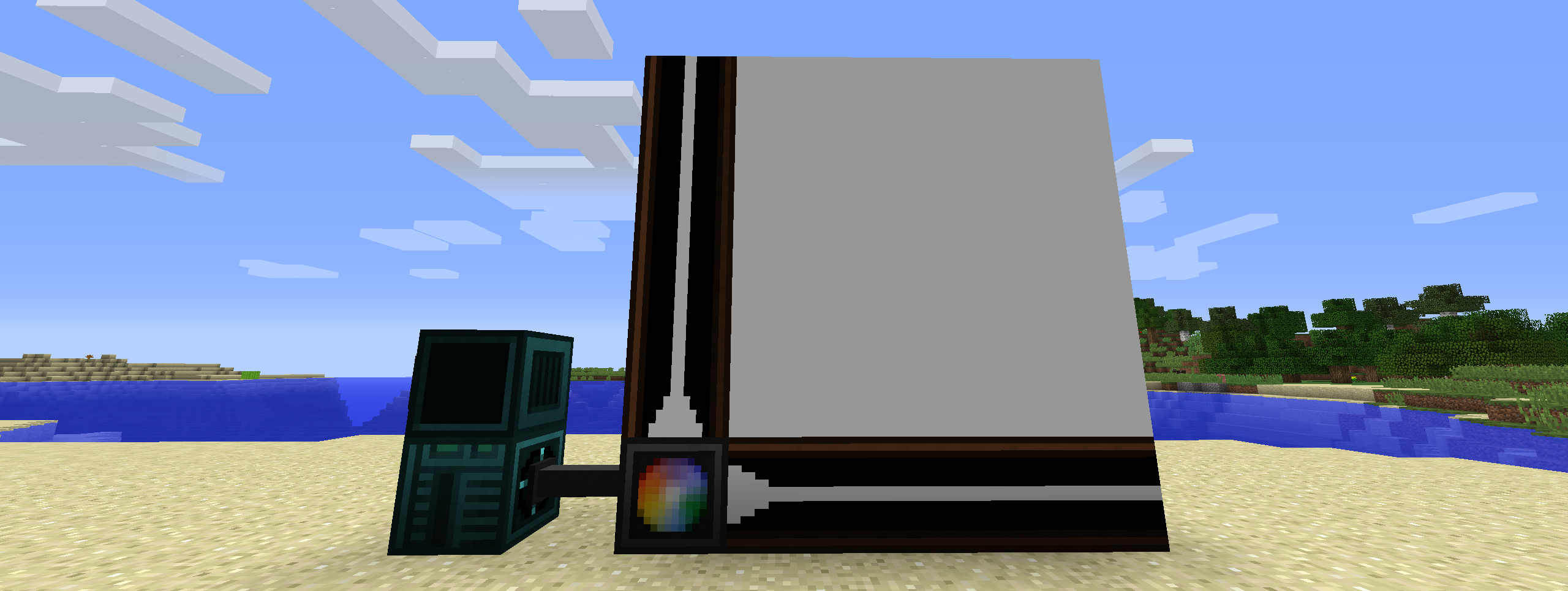# Open Lights ControllerRequires Open Computers and Open Lights

## Setup

• Drop the mod in the mods folder

## Blocks

• Open Lights Controller (4 block range)
• Open Lights Controller (8 block range)
• Open Lights Controller (16 block range)
• Open Lights Controller Border

## Usage``````-- Require component and event
component = require("component")
event = require("event")

-- Get the attached Open Lights Controller component
lc = component.openlightscontroller

-- The letters define which axis the coordinates are for
-- The value on that axis increases as it follows the border blocks away from the controller
lc.calibrate("ZY")
w,h = lc.getSize()

-- The whole grid of lights is filled with yellow and set to brightness 15
lc.fillColor(0xffff00)
lc.fillBrightness(15)

-- The light at (1,1) is set to black
lc.setColor(0x000000,1,1)

-- The lights in the area defined by (2,2) and (w,h)  are set to red
lc.fillColor(0xff0000,2,2,w,h)

-- The changes are applied to the lights
lc.apply()

-- Wait until the interrupt event
event.pull('interrupt')

-- The whole grid of lights is filled with black
lc.fillColor(0x000000)

-- The changes are applied to the lights
lc.apply()
``````

## LUA API

• `[x:number]` means these arguments are optional
• `{x:number}` means that the arguments are repeatable, or the return value is an array
Method Description
function apply():string; Applies the current cached lighting data to the lights.
function isCalibrated():bool; Checks if the light controller is calibrated.
function calibrate(direction:string):string; Calibrate light controller.
function getBorderAxes():string, string; Get border axes.
function getBorderDirections():string, string; Get active border directions.
function getSize():number, number; Get size of light grid.
function setColor(color:number, {x:number, y:number}):string; Set the light color as an RGB value. Returns the new color as an RGB hex string. Use `tonumber(value, 16)` to convert return value to a usable numeric value. The controller caches this until `apply()` is called.
function fillColor(color:number, [x1:number, y1:number, x2:number, y2:number]):string; Fills a specific region or the whole grid to a specific RGB value. Returns the new color as an RGB hex string. Use `tonumber(value, 16)` to convert return value to a usable numeric value. The controller caches this until `apply()` is called.
function setBrightness(brightness:number, {x:number, y:number}):number; Set the brightness of the light. Returns the new brightness. The controller caches this until `apply()` is called.
function fillBrightness(brightness:number, [x1:number, y1:number, x2:number, y2:number]):number; Fills a specific region or the whole grid to a specific brightness. Returns the new brightness. The controller caches this until `apply()` is called.
function getColor({x:number, y:number}):{string}; Get the light color as an RGB hex string. Use `tonumber(value, 16)` to convert return value to a usable numeric value. The controller returns the current color use `getCachedColor()` to get the currently cached color.
function getBrightness({x:number, y:number}):{number}; Get brightness of the light. The controller returns the current brightness use `getCachedBrightness()` to get the currently cached brightness.
function getCachedColor({x:number, y:number}):{string}; Get the light color as an RGB hex string. Use `tonumber(value, 16)` to convert return value to a usable numeric value. Use `getColor()` to get the current color of the light.
function getCachedBrightness({x:number, y:number}):{number}; Get brightness of the light. Use `getBrightness()` to get the current brightness of the light.
function getMaximumBorderSize():number; Get the maximum border size. This changes depending on the tier of the light controller.